【deque容器系列一】基于STL源码分析deque容器整体实现及内存结构#### 1. deque容器整体源码实现介绍

deque容器是stl中顺序容器的一种，之前已经介绍过array和vector了，今天介绍deque容器，deque的本质是一个类模板，它的声明位于头文件`bits/stl_deque.h`，实现位于`bits/deque.tcc`，接下来我们就围绕这两个文件来介绍一下deque容器的实现原理。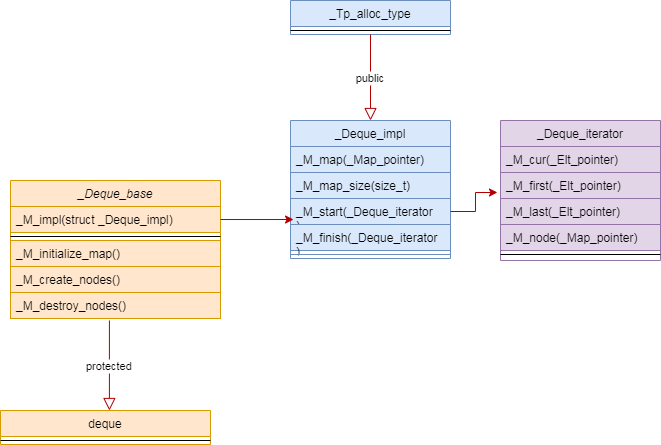• deque容器保护继承于类模板`_Deque_base`，也就是`_Deque_base`是deque的基类，并且内存分配和释放都是通过基类来完成的；
• 容器首地址和迭代器等保存在结构体成员变量`_M_impl`中，它继承于别名类型`_Tp_alloc_type`，最终的内存分配其实就是通过它完成的；
• deque容器使用了它自己的迭代器`_Deque_iterator`，没有直接使用stl中的公共迭代器，且迭代器里面保存了当前地址、首地址、尾地址以及当前节点。

``````//这里_Tp是模板类型
#if __cplusplus < 201103L
typedef _Deque_iterator<_Tp, _Tp&, _Tp*>         iterator;
typedef _Deque_iterator<_Tp, const _Tp&, const _Tp*> const_iterator;
typedef _Tp*                     _Elt_pointer;
typedef _Tp**                    _Map_pointer;
#else
private:
template<typename _Up>
using __ptr_to = typename pointer_traits<_Ptr>::template rebind<_Up>;
template<typename _CvTp>
using __iter = _Deque_iterator<_Tp, _CvTp&, __ptr_to<_CvTp>>;
public:
typedef __iter<_Tp>        iterator;
typedef __iter<const _Tp>        const_iterator;
typedef __ptr_to<_Tp>        _Elt_pointer;
typedef __ptr_to<_Elt_pointer>    _Map_pointer;
#endif``````

#### 2. deque容器构造时内存结构是怎样的

``````//构造一个大小为n的deque容器，容器中所有元素的值为value，__a是默认分配器
deque(size_type __n, const value_type& __value, const allocator_type& __a = allocator_type())
: _Base(__a, __n)
{ _M_fill_initialize(__value); }``````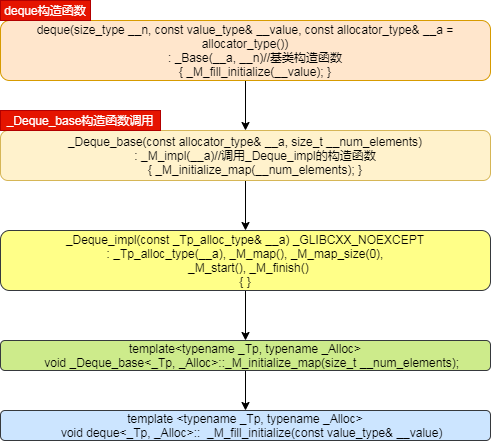``````//deque容器初始化元素个数
template<typename _Tp, typename _Alloc>
void _Deque_base<_Tp, _Alloc>::_M_initialize_map(size_t __num_elements)
{
//如果sizeof(_Tp)<512，那么__deque_buf_size返回512/sizeof(_Tp)，否则返回1，_Tp是容器的元素类型，所以这里返回的是一个默认块内存的元素数量，也就是一个buffer里面包含的元素个数，后续以buffer代指一块内存
//__num_nodes = 元素个数/块内存元素数量 + 1，得到的是总共有多少个buffer，为什么要加1，防止有余数，所以需要加1
const size_t __num_nodes = (__num_elements/ __deque_buf_size(sizeof(_Tp))
+ 1);
//_S_initial_map_size默认为8，根据max调用得到最终的块内存个数
this->_M_impl._M_map_size = std::max((size_t) _S_initial_map_size,
size_t(__num_nodes + 2));
//这里根据buffer类型和buffer个数申请动态内存，说白了这里申请节点的动态内存，并没有申请真正保存元素的块内存的动态空间
this->_M_impl._M_map = _M_allocate_map(this->_M_impl._M_map_size);
//根据以下两行可知，_M_map的第一个和最后一个节点其实是保留的，暂时不会使用
_Map_pointer __nstart = (this->_M_impl._M_map
+ (this->_M_impl._M_map_size - __num_nodes) / 2);
_Map_pointer __nfinish = __nstart + __num_nodes;
__try
{
//该函数根据节点循环对每一块buffer申请动态空间，一个节点指向一个buffer
_M_create_nodes(__nstart, __nfinish); }
__catch(...)
{
_M_deallocate_map(this->_M_impl._M_map, this->_M_impl._M_map_size);
this->_M_impl._M_map = _Map_pointer();
this->_M_impl._M_map_size = 0;
__throw_exception_again;
}
//_M_set_node对节点所对应的位置和迭代器位置进行初始化，并使用成员变量保存节点开始和结束位置
this->_M_impl._M_start._M_set_node(__nstart);
this->_M_impl._M_finish._M_set_node(__nfinish - 1);
this->_M_impl._M_start._M_cur = _M_impl._M_start._M_first;
this->_M_impl._M_finish._M_cur = (this->_M_impl._M_finish._M_first
+ __num_elements
% __deque_buf_size(sizeof(_Tp)));
}``````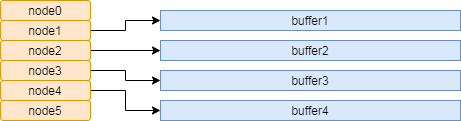``````template <typename _Tp, typename _Alloc>
void
deque<_Tp, _Alloc>::
_M_fill_initialize(const value_type& __value)
{
_Map_pointer __cur;
__try
{
//循环填充容器每一个元素的值为__value
for (__cur = this->_M_impl._M_start._M_node;
__cur < this->_M_impl._M_finish._M_node;
++__cur)
//__uninitialized_fill_a函数会根据值对每一个元素进行构造，它其实相当于一个placement new的作用
std::__uninitialized_fill_a(*__cur, *__cur + _S_buffer_size(),
__value, _M_get_Tp_allocator());
std::__uninitialized_fill_a(this->_M_impl._M_finish._M_first,
this->_M_impl._M_finish._M_cur,
__value, _M_get_Tp_allocator());
}
__catch(...)
{
std::_Destroy(this->_M_impl._M_start, iterator(*__cur, __cur),
_M_get_Tp_allocator());
__throw_exception_again;
}
}``````

``````#include <deque>

int main()
{
std::deque<int> deq(1024, 100);
return 0;
}``````blmius
1年前
MySQL:[Err] 1292 - Incorrect datetime value: ‘0000-00-00 00:00:00‘ for column ‘CREATE_TIME‘ at row 1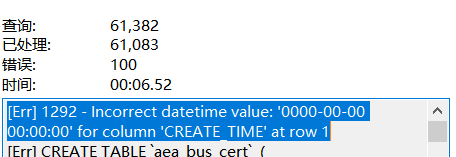cpp加油站
1年前
c++11增加的变参数模板，今天总算整明白了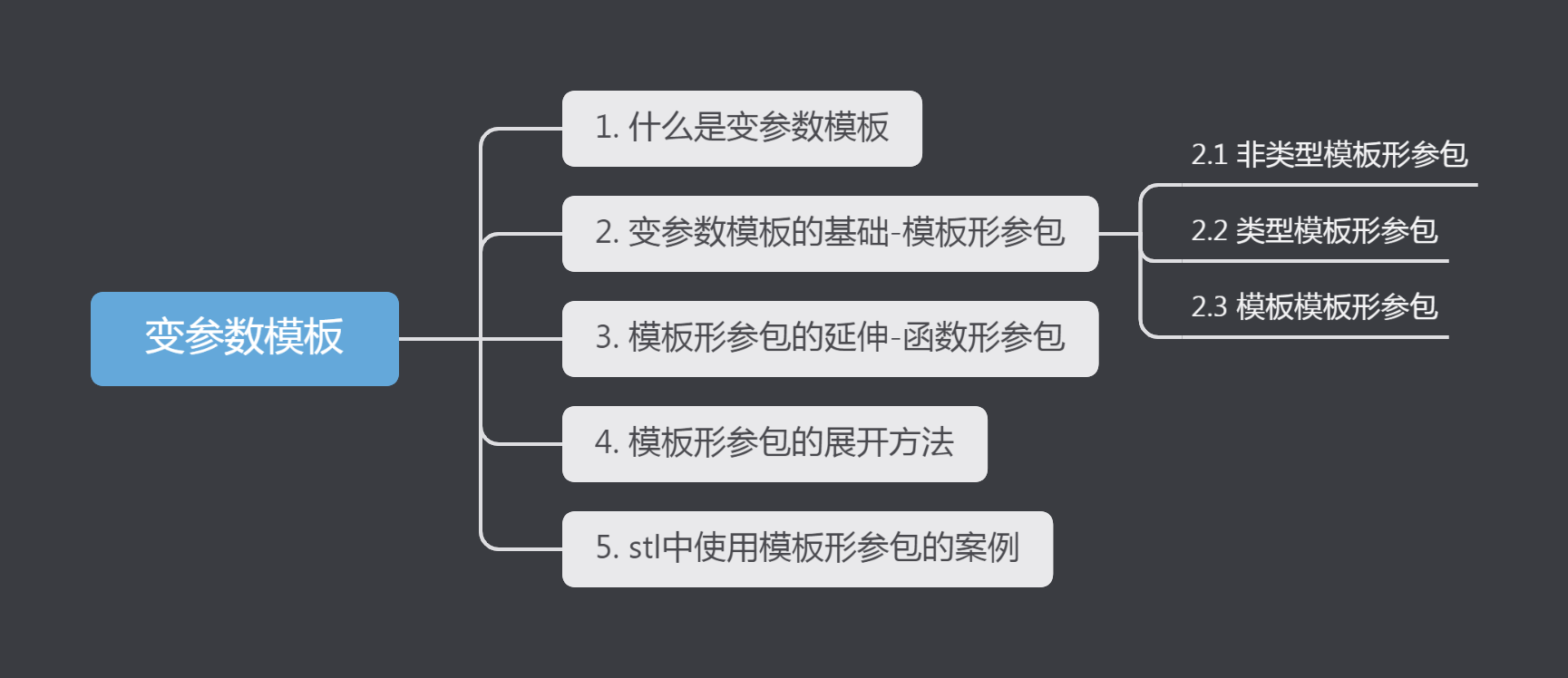Jacquelyn38
2年前
2020年前端实用代码段，为你的工作保驾护航cpp加油站
1年前
【deque容器系列二】基于STL源码分析deque容器插入和删除时内存都是怎么变动的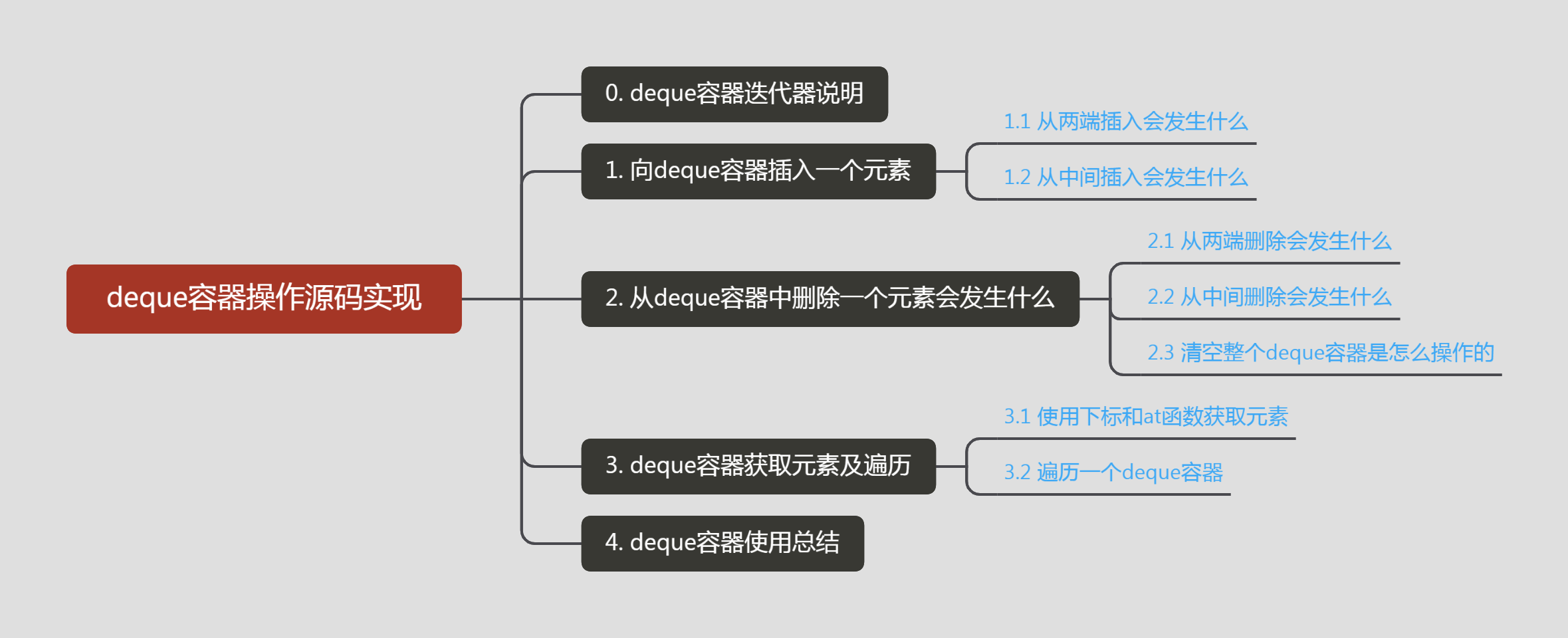cpp加油站
1年前
【STL源码拆解】基于源码分析forward_lsit容器实现(详细!)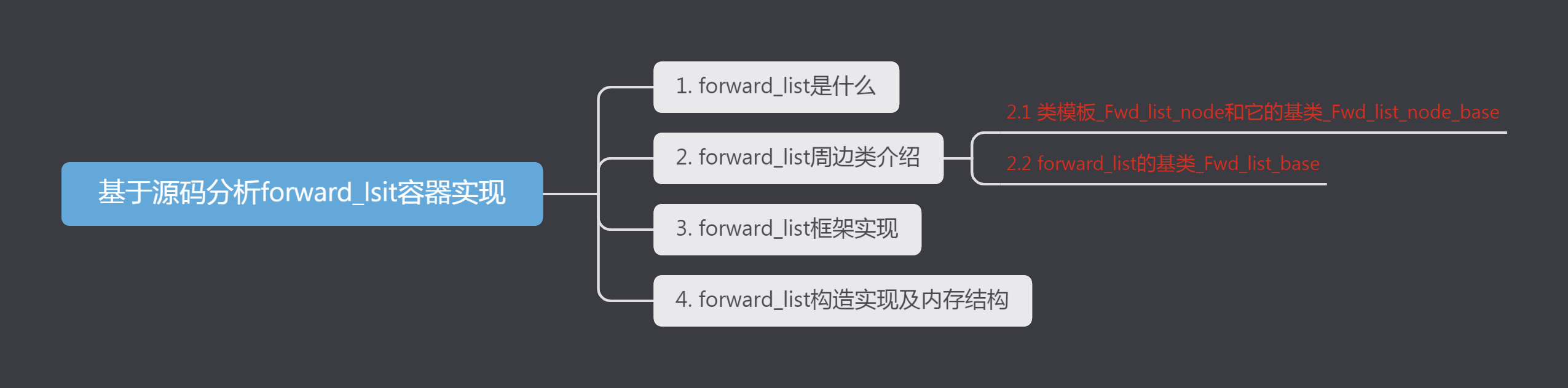helloworld_34035044
11个月前

​在我们用皕杰报表工具设计填报报表时，如何在新增行里自动增加id呢？能新增整数排序id吗？目前可以在新增行里自动增加id，但只能用uuid函数增加UUID编码，不能新增整数排序id。uuid函数说明：获取一个UUID，可以在填报表中用来创建数据ID语法：uuid()或uuid(sep)参数说明：sep布尔值，生成的uuid中是否包含分隔符''，缺省为Stella981
1年前
KVM调整cpu和内存Easter79
1年前Stella981
1年前
C++STL容器deque
deque简介deque属于序列式容器，和vector十分相似，采用dynamicarray来管理元素，提供随机访问，但是deque的dynamicarray头尾两端都开放，可以在头尾两端快速安插和删除。!(https://img2018.cnblogs.com/blog/1520224/201902/15202242019Wesley13
1年前
MySQL部分从库上面因为大量的临时表tmp_table造成慢查询cpp加油站
Lv1

16

5

10